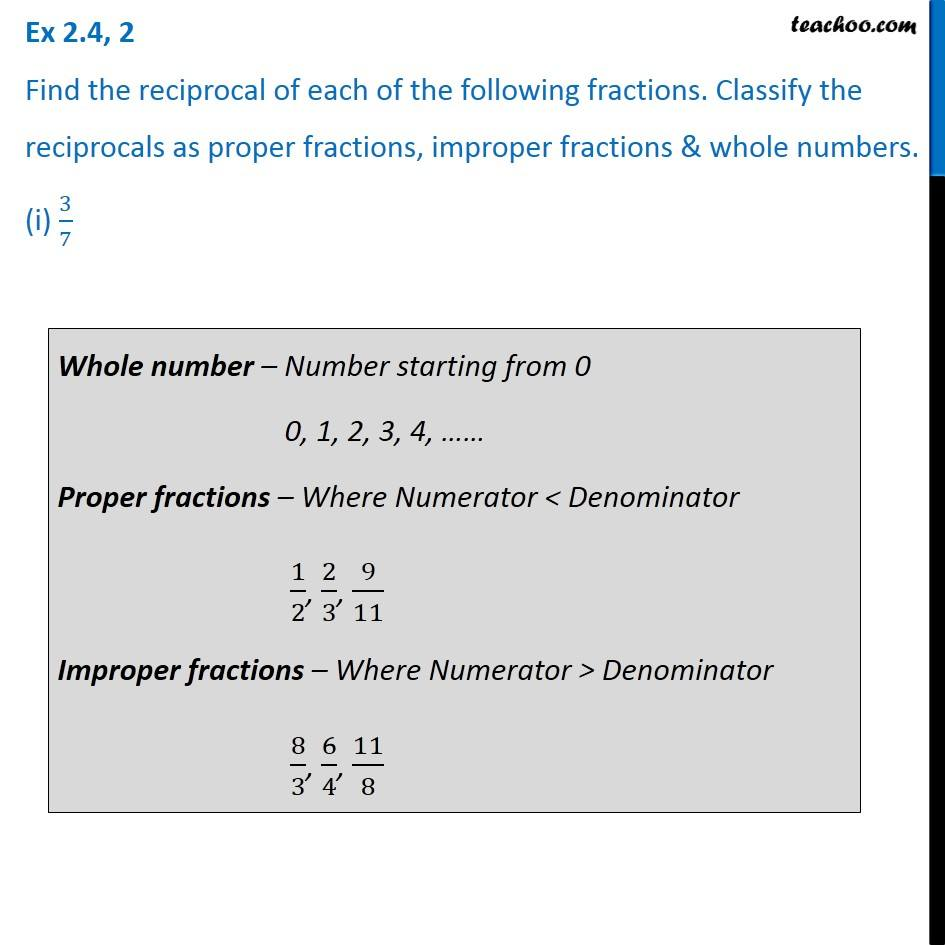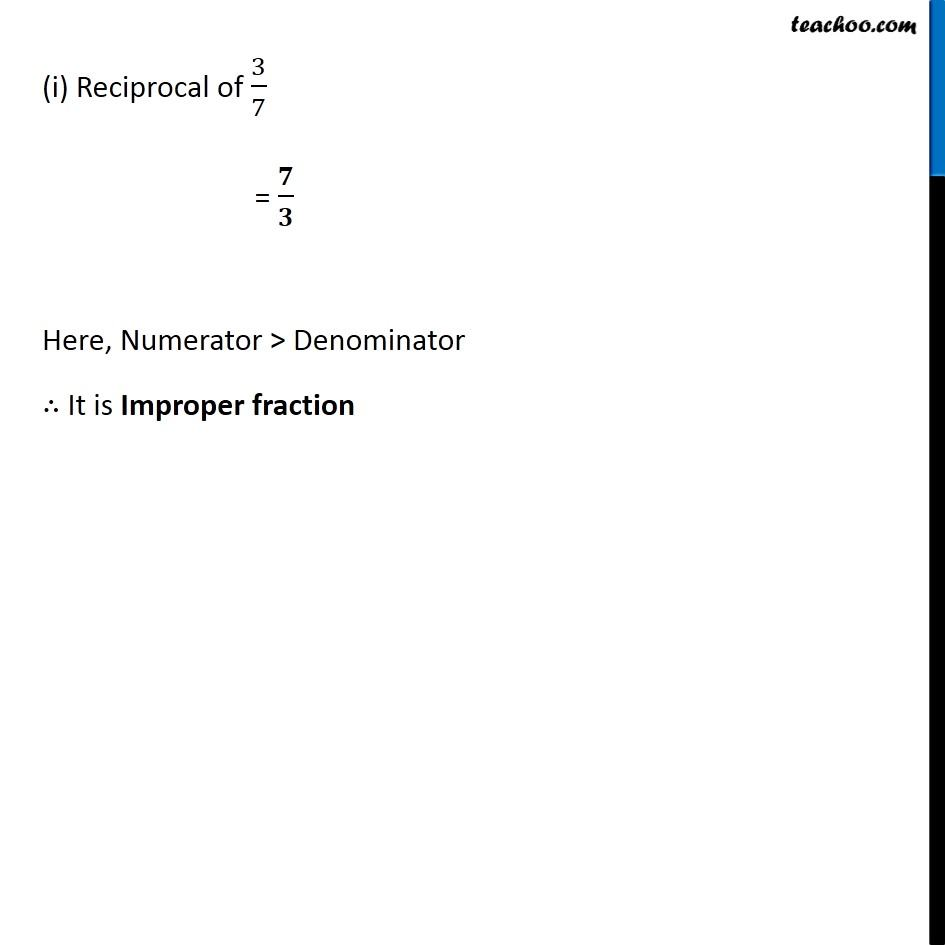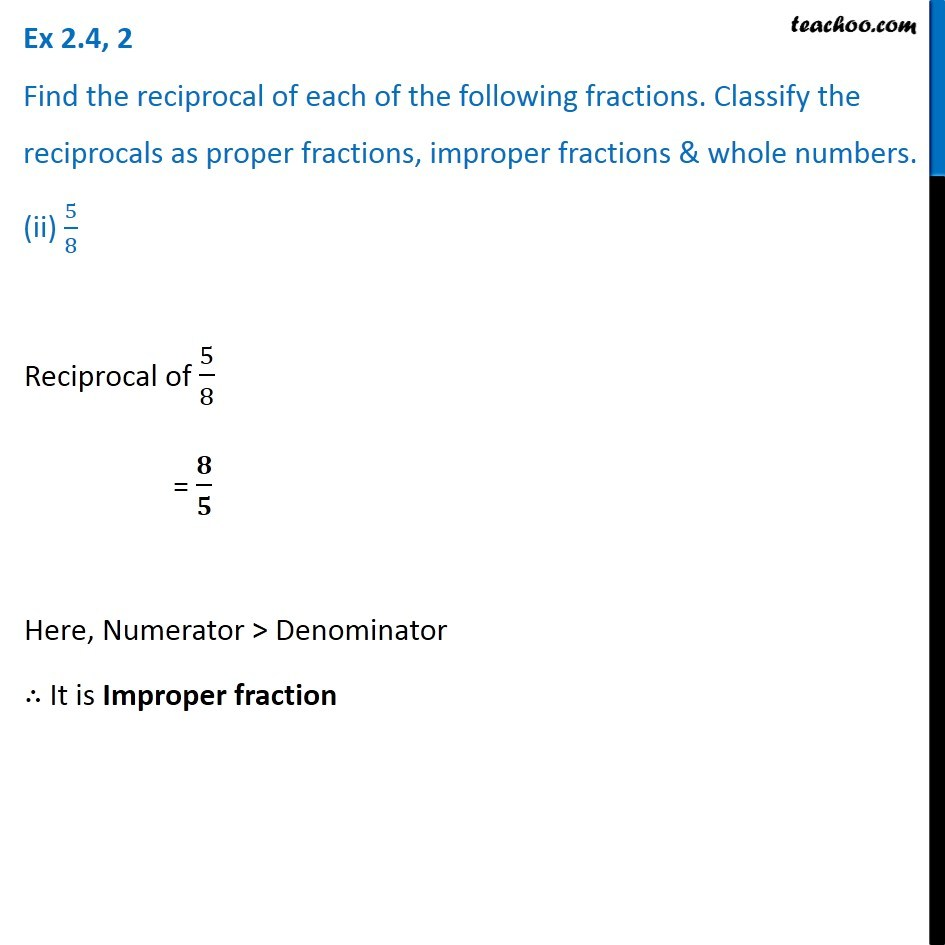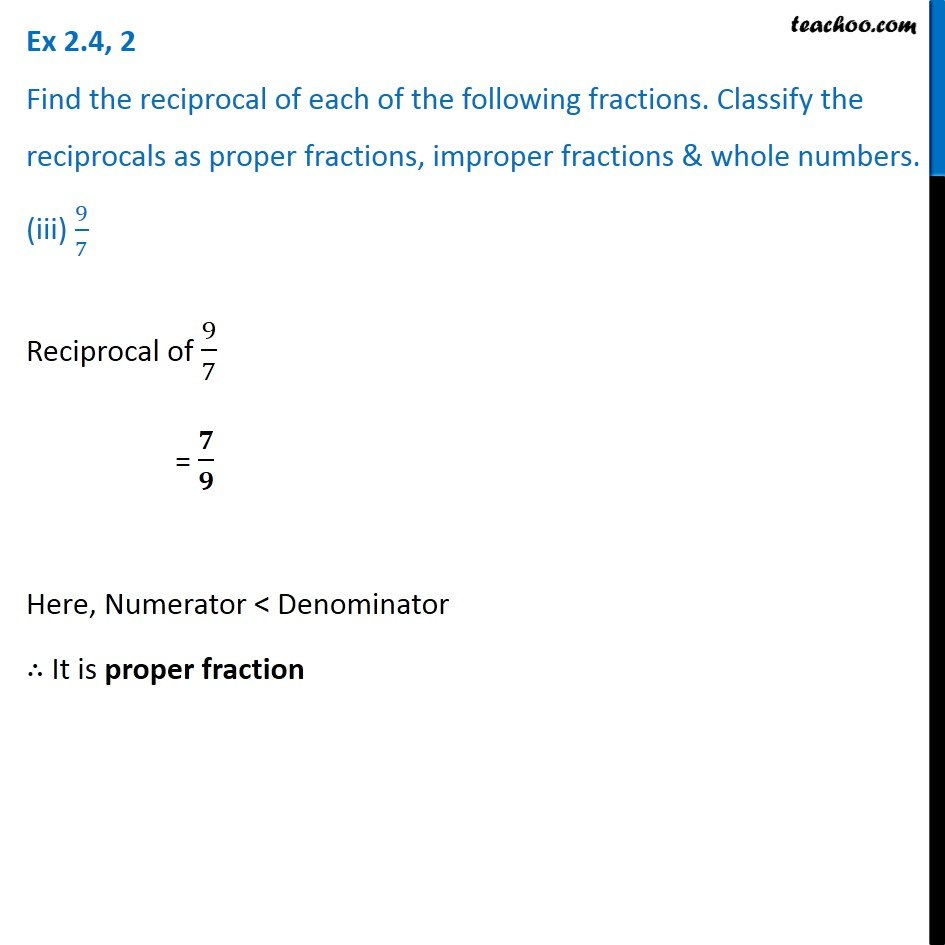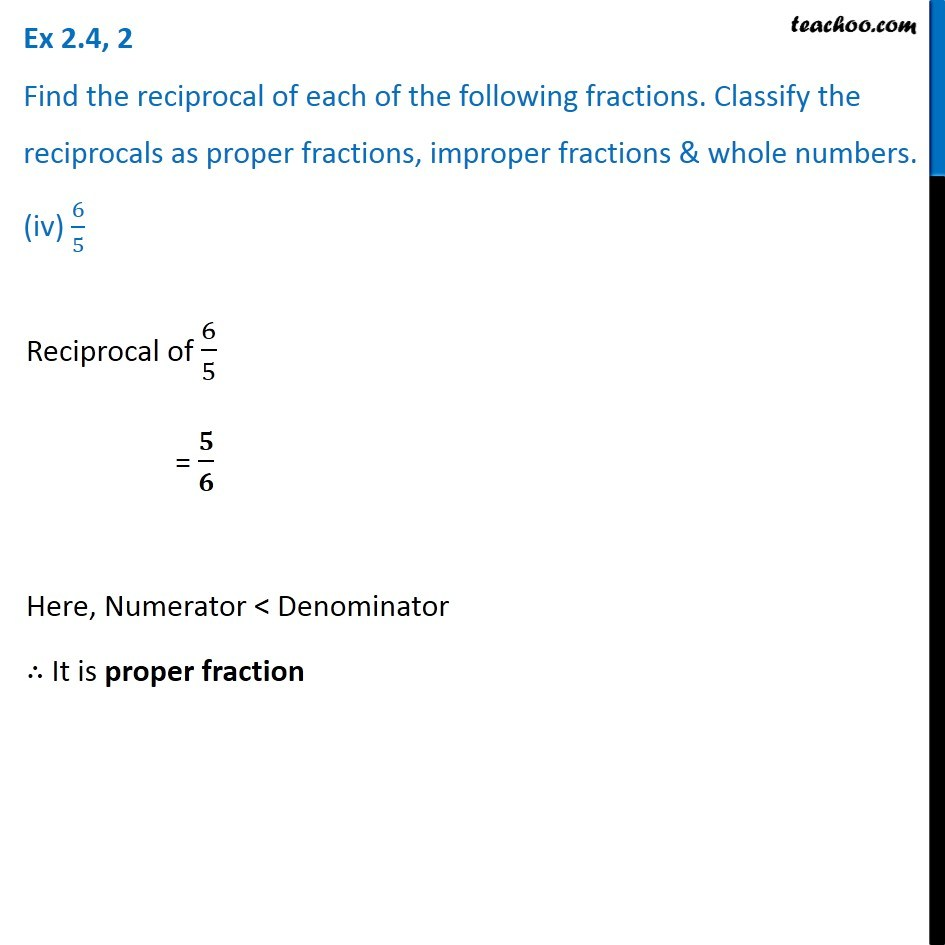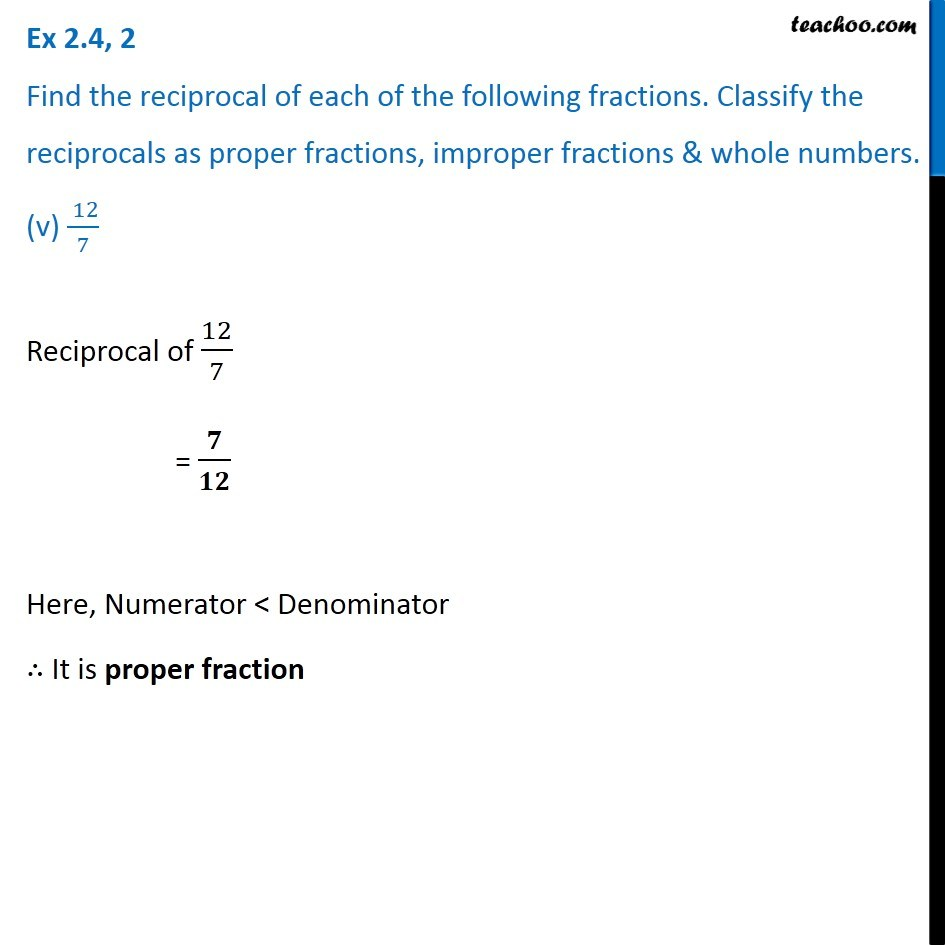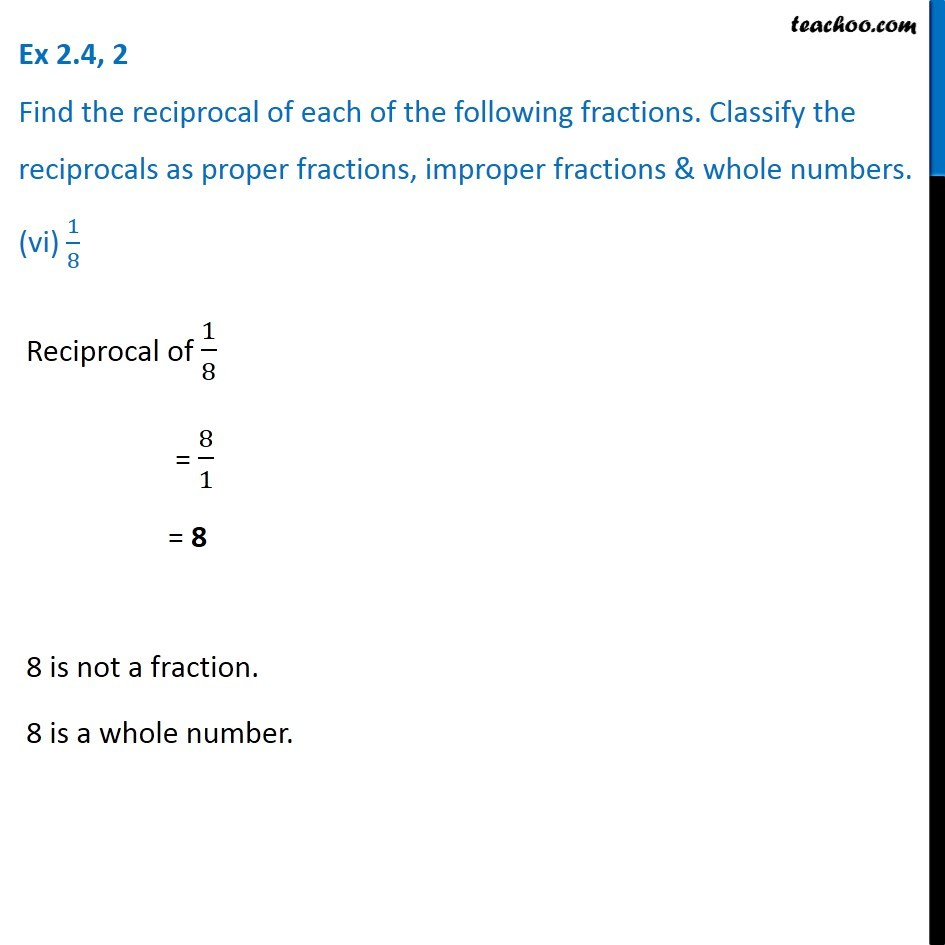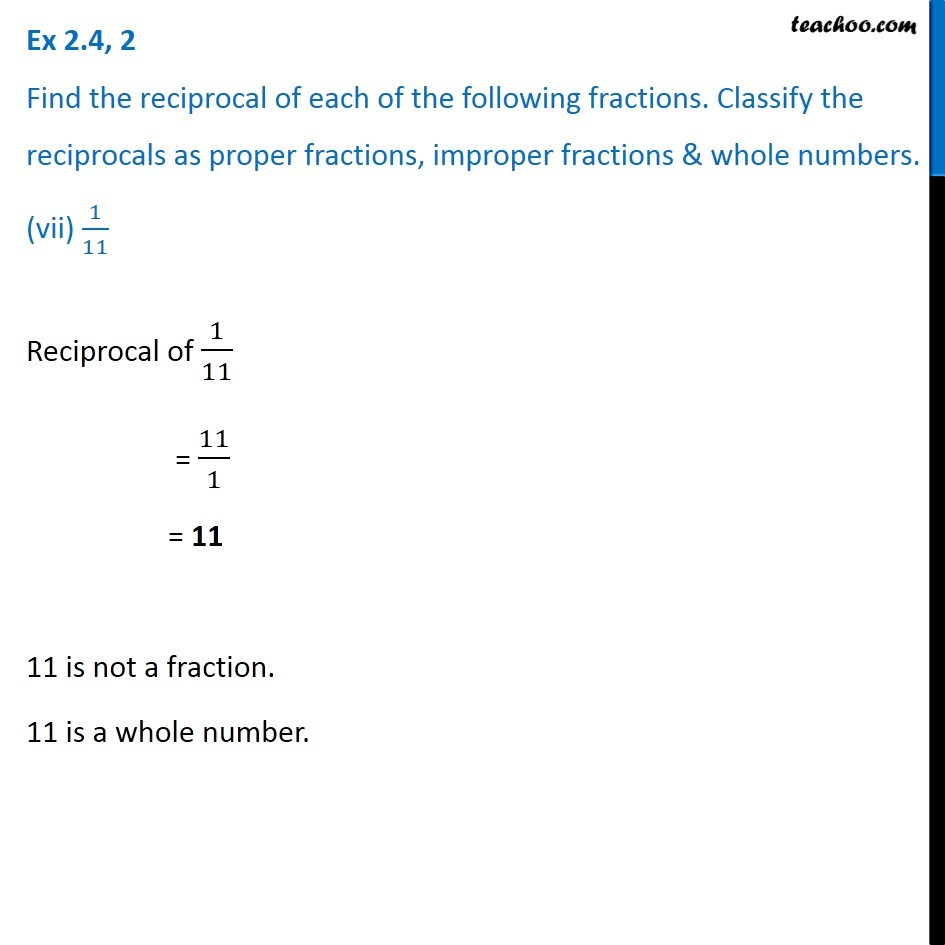Subscribe to our Youtube Channel - https://you.tube/teachoo

1. Chapter 2 Class 7 Fractions and Decimals
2. Serial order wise
3. Ex 2.4

Transcript

Ex 2.4, 2 Find the reciprocal of each of the following fractions. Classify the reciprocals as proper fractions, improper fractions & whole numbers. (i) 3/7Whole number – Number starting from 0 0, 1, 2, 3, 4, …… Proper fractions – Where Numerator < Denominator 1/2, 2/3, 9/11 Improper fractions – Where Numerator > Denominator 8/3, 6/4, 11/8 (i) Reciprocal of 3/7 = 𝟕/𝟑 Here, Numerator > Denominator ∴ It is Improper fraction Ex 2.4, 2 Find the reciprocal of each of the following fractions. Classify the reciprocals as proper fractions, improper fractions & whole numbers. (ii) 5/8Reciprocal of 5/8 = 𝟖/𝟓 Here, Numerator > Denominator ∴ It is Improper fraction Ex 2.4, 2 Find the reciprocal of each of the following fractions. Classify the reciprocals as proper fractions, improper fractions & whole numbers. (iii) 9/7Reciprocal of 9/7 = 𝟕/𝟗 Here, Numerator < Denominator ∴ It is proper fraction Ex 2.4, 2 Find the reciprocal of each of the following fractions. Classify the reciprocals as proper fractions, improper fractions & whole numbers. (iv) 6/5Reciprocal of 6/5 = 𝟓/𝟔 Here, Numerator < Denominator ∴ It is proper fraction Ex 2.4, 2 Find the reciprocal of each of the following fractions. Classify the reciprocals as proper fractions, improper fractions & whole numbers. (v) ( 12)/7Reciprocal of 12/7 = 𝟕/𝟏𝟐 Here, Numerator < Denominator ∴ It is proper fraction Ex 2.4, 2 Find the reciprocal of each of the following fractions. Classify the reciprocals as proper fractions, improper fractions & whole numbers. (vi) 1/8Reciprocal of 1/8 = 8/1 = 8 8 is not a fraction. 8 is a whole number. Ex 2.4, 2 Find the reciprocal of each of the following fractions. Classify the reciprocals as proper fractions, improper fractions & whole numbers. (vii) 1/11Reciprocal of 1/11 = 11/1 = 11 11 is not a fraction. 11 is a whole number.

Ex 2.4